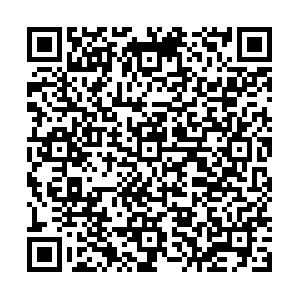EI、Scopus 收录

## 留言板引用本文: 郭子文, 章公也, 糜长稳. 磁驱动中心对称挠曲电夹层板力电耦合性能分析. 力学学报, 2023, 55(7): 1-9Guo Ziwen, Zhang Gongye, Mi Changwen. On the magnetically induced electromechanical coupling of centrosymmetric flexoelectric sandwich plate. Chinese Journal of Theoretical and Applied Mechanics, 2023, 55(7): 1-9 doi: 10.6052/0459-1879-23-103
 Citation: Guo Ziwen, Zhang Gongye, Mi Changwen. On the magnetically induced electromechanical coupling of centrosymmetric flexoelectric sandwich plate. Chinese Journal of Theoretical and Applied Mechanics, 2023, 55(7): 1-9• 中图分类号: TB381

## ON THE MAGNETICALLY INDUCED ELECTROMECHANICAL COUPLING OF CENTROSYMMETRIC FLEXOELECTRIC SANDWICH PLATE

• 摘要: 现代工业的发展对材料性能和结构尺寸提出更高的要求, 机电器件的设计越来越偏向于小型化、高频化和智能化. 最新研究成果表明, 磁电耦合复合材料不仅能够以较强的磁电转换效率实现磁能、机械能和电能之间的相互转换, 还可以避免结构与机械驱动源的直接接触, 实现非接触调控, 这对于制备多功能微纳米器件具有重要意义. 文章基于Mindlin所发展的多物理场结构理论分析方法, 结合宏观压磁理论和偶应力挠曲电理论, 研究由单个挠曲电电介质层和两个对称压磁层构成的三明治型夹层板在外部横向磁场驱动下的动态力电耦合响应, 其中通过引入曲率将经典力电耦合理论拓宽到中心对称材料. 夹层板在正弦型全局磁场和均布局部磁场驱动下的动态数值算例表明: 位移和电势具有一定的频率依赖性, 当激振频率达到固有频率时, 振幅达到最大值; 此外, 对称式驱动压磁层分布方式趋于提高多层复合板的力电耦合性能. 文章理论模型和研究结果可为磁控机电器件的优化设计提供新的改进思路.

• 图  1  单层板及采用的坐标系

Figure  1.  A single-layer plate and coordinate system

图  2  磁场作用下的夹层板模型

Figure  2.  A sandwich plate model under a magnetic field

图  3  横向磁场驱动下夹层板的边界配置

Figure  3.  Boundary configuration of sandwich plate under a transverse magnetic field

图  4  全局磁场驱动下的一阶电势幅频关系对比

Figure  4.  Comparison of amplitude-frequency curve of first-order potential under the global magnetic field

图  5  局部驱动磁场作用区域(灰色区域)

Figure  5.  Local magnetic field area (grey area)

图  6  局部磁场驱动下夹层板的挠度幅频关系

Figure  6.  Deflection amplitude-frequency curve of the sandwich plate under local magnetic field

图  7  局部磁场驱动下夹层板的前两阶挠度、转角和一阶电势振型

Figure  7.  The first two order deflection, rotation angle and first-order potential mode of the sandwich plate under the local magnetic field

7  局部磁场驱动下夹层板的前两阶挠度、转角和一阶电势振型 (续)

7.  The first two order deflection, rotation angle and first-order potential mode of the sandwich plate under the local magnetic field (continued)

表  1  CoFe2O4和Si的材料参数

Table  1.   Material parameters of CoFe2O4 and Si

 Property/Unit CoFe2O4 Si elastic constants/GPa ${ {C'_{11}} } = 286 \\$ ${ {C'_{1{\text{2} }} } } = {\text{173} }$ ${C_{11}} = 165.7$ ${ {C'_{13}} } = 170 \\$ ${C_{12}} = 63.9 \\$ ${ {C'_{33} }} = 269.5 \\$ ${C_{44}} = 79.56$ ${ {C'_{44}} } = 45.3$ dielectric constants/(C2·(N·m2)−1)×10−9 ${ {s'_{11}} } = 0.{\text{08} } \\$ ${s_{11} } = 0.1035$ ${ {s'_{33}} } = 0.{\text{093} }$ piezomagnetic constants/(N·(A·m)−1) ${ {h'_{31}} } = 580.3$ — ${ {h'_{33} }} = 699.7$ flexoelectric coefficient/(nC·m−1) — ${f_{16}} = 0.4$ mass density/(kg·m−3) $\rho ' = 5300$ $\rho = 2332$
•点击查看大图
##### 计量
• 文章访问数:  77
• HTML全文浏览量:  31
• PDF下载量:  19
• 被引次数: 0
##### 出版历程
• 收稿日期:  2023-03-21
• 录用日期:  2023-05-09
• 网络出版日期:  2023-05-10

### 目录/下载:  全尺寸图片 幻灯片
• 分享
• 用微信扫码二维码

分享至好友和朋友圈